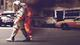• # ErrUchIsDown - code seems to pass...

I would like to give some feedback about ...

HTTP_USER_AGENT:

```Mozilla/5.0 (Macintosh; Intel Mac OS X 10_10_5) AppleWebKit/537.36 (KHTML, like Gecko) Chrome/50.0.2661.94 Safari/537.36
```

My Code running a DFS recursion seems to pass locally with python3.4 on mac running Captain, but in the browser it is getting ErrUchIsDown when it says loading(14) or loading(28). Maybe someone has seen this before or maybe the code just isn't actually working or the recursion is going too deep?? I'm not sure.

Code: def find_cycle(cons): nodes = list(set([each for con in cons for each in con])) cycles = []

```def getpaths(node, seen):
paths = []
for con in cons:
if node in con and con not in seen:
newnode = con if con == node else con
if sum(x.count(node) for x in seen) < 2 and sum(x.count(newnode) for x in seen) < 2:
paths.append(con)
return paths

def followcycle(node, seen):
paths = getpaths(node, seen)
for path in paths:
newnode = path if path != node else path
cycles.append(followcycle(newnode, seen + [path]))
return seen

for node in nodes:
followcycle(node, [])

largest = []
for cycle in cycles:
if len(cycle) > 2:
if cycle == cycle[-1] or cycle == cycle[-1]:
if len(cycle) > len(largest):
largest = cycle

myl = []
[myl.append(x) for i in largest for x in i if x not in myl]
return myl + [myl] if len(myl) else []
```15